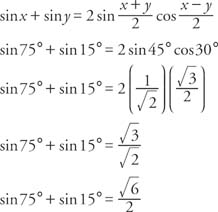## Product‐Sum and Sum‐Product Identities

The process of converting sums into products or products into sums can make a difference between an easy solution to a problem and no solution at all. Two sets of identities can be derived from the sum and difference identities that help in this conversion. The following set of identities is known as the product‐sum identities.These identities are valid for degree or radian measure whenever both sides of the identity are defined.

Example 1: Verify that sin α cos β =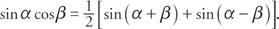Start by adding the sum and difference identities for the sine.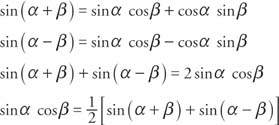The other three product‐sum identities can be verified by adding or subtracting other sum and difference identities.

Example 2: Write cos 3 x cos 2 x as a sum.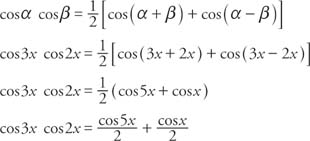Alternate forms of the product‐sum identities are the sum‐product identities.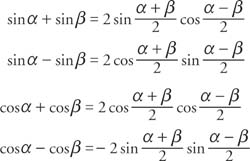These identities are valid for degree or radian measure whenever both sides of the identity are defined.

Example 3:Solve for α by adding the following two equations and then dividing by 2. Solve for β by subtracting the two equations and then dividing by 2.Example 4: Write the difference cos 8α − cos 2 α as a product.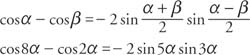Example 5: Find the exact value of sin 75° + sin 15°.